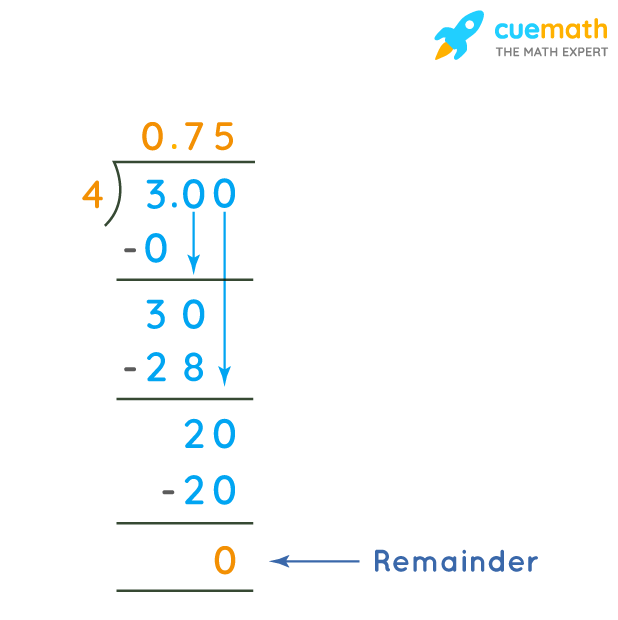# 3/4 in Decimal Form

## Question: What is 3/4 in decimal form?

Both fractions and decimals are just two ways to represent the same numbers.

## Answer: 3/4 as a decimal is equal to 0.75

Let's convert the given fraction to a decimal number.

## Explanation:

To convert any fraction to decimal form, we just need to divide its numerator by denominator.

Here the fraction is 3/4 which means we need to perform 3 ÷ 4.### Alternate Method:

It is difficult to perform a division when a dividend is less than the divisor.

So, we re-write 3/4 as 3 × (1/4)

• 1/4 = [(100/4) ÷ 100].
• 100/4 = 25
• 25 ÷ 100 = 0.25
• So, 1/4 = 0.25.

Therefore, 3/4 = 3 × (1/4) = 3 × 0.25 = 0.75.